## The second-moment method

### Quick description

One can often draw interesting consequences from the information that a certain random variable is almost constant. In many contexts, a good definition of "is almost constant" is "has small variance". The reason this is a good definition is that of all measures of near constancy, the variance is arguably the most natural and is often the easiest to calculate. This is best illustrated with some examples.

### Prerequisites

Two equivalent definitions of variance of a random variable, basic definitions concerning sets, graphs etc.

### Example 1

This example may seem slightly artificial, but in fact it is an important argument in additive combinatorics.

Let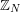denote the set of integers mod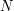. Let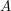be a subset ofof size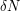. Define an additive quadruple into be a quadruple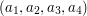of elements ofwith the property that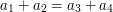. Suppose that the number of additive quadruples of elements ofis at most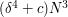. We shall show, providedis small enough, thatmust contain an arithmetic progression of length 3. More precisely, we shall show that there must existand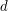such that neithernor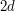is equal to 0 mod, and,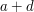and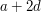all belong to.

To do this, we define a function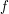as follows: for each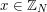,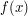is the number of ways of writingas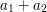with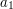and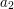belonging to. The following facts aboutare easy to see. First,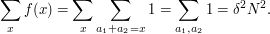Secondly,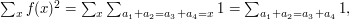which is the number of additive quadruples of elements of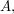which we are assuming to be at most.

It follows that the average value ofis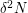and the average value of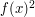is at most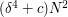. Therefore, the variance of, which is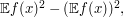is at most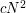.

We now use Chebyshev's inequality, which tells us that if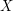is any random variable, then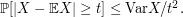Here is a quick proof of the inequality, which is also discussed in the article Bounding probabilities by expectations. We know that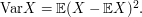This is certainly at least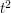times the probability that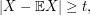so the inequality follows. For us the random variable is, whereis chosen randomly and uniformly from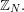Applying Chebyshev's inequality, we find that the proportion ofsuch that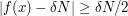is at most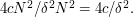Therefore, if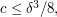the number ofsuch that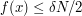is at most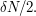Nowhaselements, so there must be at least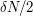elements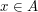such that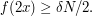This implies that the number of triples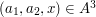such that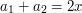is at least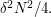Each such triple corresponds to an arithmetic progression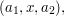as long as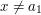and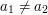. The number of these degenerate triples is at most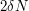, so as long asis sufficiently large (it is enough if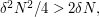which is the case if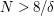) there must be non-degenerate ones, as claimed.

### General discussion

Let us think briefly about how the above argument might have been discovered. The idea was to find some fairly general condition on a set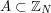that would guarantee thatcontained an arithmetic progression of length 3. Nowwill certainly contain an arithmetic progression of length 3 if we can find somesuch that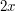can be written in many ways as, withandalso belonging to. And this will certainly be the case if the functiondefined above is close to its average valuefor more than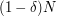values of. Speaking more loosely, we would liketo be roughly constant. So it is natural to look at the variance of, which turns out to be closely related to the number of additive quadruples in the set.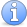Incomplete This article is incomplete. Several more examples wanted.